### IMO Shortlist 2002 problem G2

Kvaliteta:
Avg: 0,0
Težina:
Avg: 6,0
Let$ABC$ be a triangle for which there exists an interior point$F$ such that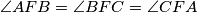$\angle AFB=\angle BFC=\angle CFA$. Let the lines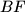$BF$ and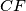$CF$ meet the sides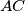$AC$ and$AB$ at$D$ and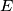$E$ respectively. Prove that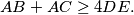Izvor: Međunarodna matematička olimpijada, shortlist 2002